# Texas Go Math Grade 4 Lesson 3.3 Answer Key Simplest Form

Refer to our Texas Go Math Grade 4 Answer Key Pdf to score good marks in the exams. Test yourself by practicing the problems from Texas Go Math Grade 4 Lesson 3.3 Answer Key Simplest Form.

## Texas Go Math Grade 4 Lesson 3.3 Answer Key Simplest Form

Essential Question

How can you write o fraction as on equivalent fraction in simplest form?

Unlock the Problem

Vicki bought an ice cream cake cut into 6 equal pieces. Vicki, Margo, and Elena each took 2 pieces of the cake home. Vicki says she and each of her friends took $$\frac{1}{3}$$ of the cake home. Is Vicki correct?

• Into how many pieces was the cake cut?

• How many pieces did each girl take?

Activity

Materials: color pencils

STEP 1 Use a blue pencil to shade the pieces Vicki took home.
STEP 2 Use a red pencil to shade the pieces Margo took home.
STEP 3 Use a yellow pencil to shade the pieces Elena took home.The cake is divided into ________ equal-size pieces. The 3 colors on the model show how to combine sixth-size pieces to make _________ equal third-size pieces.

So, Vicki is correct. Vicki, Margo, and Elena each took $$\frac{}{}$$ of the cake home.

What if Vicki took 3 pieces of cake home and Elena took 3 pieces of cake home. How could you combine the pieces to write a fraction that represents the part each friend took home? Explain.

Math Talk

Mathematical Processes
Compare the models for $$\frac{2}{6}$$ and $$\frac{1}{3}$$. Explain how the sizes the parts are related.

One Way Use models to write an equivalent fraction in simplest form.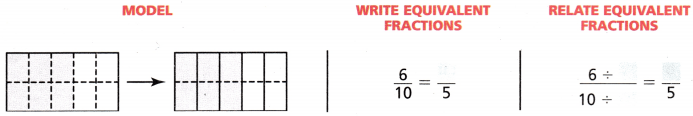To simplify $$\frac{6}{10}$$, you can combine tenth-size parts into equal groups with 2 parts each.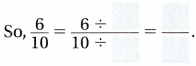Another Way use common factors to write $$\frac{6}{10}$$ in simplest form.

A fraction is in simplest form when 1 is the only factor that the numerator and denominator have in common. The parts of the whole cannot be combined into fewer equal-size parts to show the same fraction.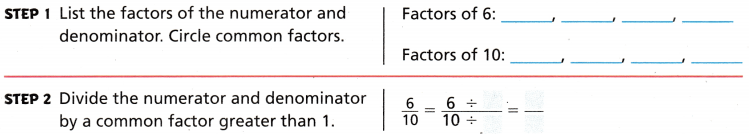So, $$\frac{6}{10}$$ written in simplest form is __________

Share and Show

Question 1.
Write $$\frac{8}{10}$$ in simplest form.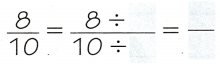Math Talk

Mathematical Processes
Explain how you know a fraction is in simplest form.

Write the fraction in simplest form.

$$\frac{6}{12}$$

Question 3.
$$\frac{2}{10}$$

Question 4.
$$\frac{6}{8}$$

Question 5.
$$\frac{4}{6}$$

Problem Solving

Use the map for 6 – 8.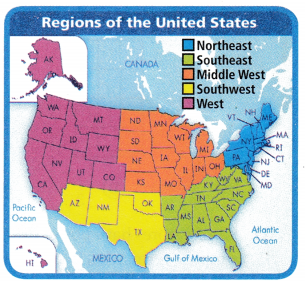Question 6.
Use Diagrams What fraction of the states in the Southwest region share a border with Mexico? Is this fraction in simplest form?

Question 7.
H.O.T. What’s the Question?
$$\frac{1}{3}$$ of the states in this region are on the Gulf of Mexico.

Multi-Step What fraction of states are in the Southwest and Southeast regions combined? Write the fraction in simplest form.

Question 9.
H.O.T. Sense or Nonsense?
Pete says that to write $$\frac{4}{6}$$ as $$\frac{2}{3}$$, you combine pieces, but to write $$\frac{4}{6}$$ as $$\frac{8}{12}$$, you break apart pieces. Does this make sense? Explain.

Question 10.
Use Diagrams Emily’s pet cat has lived with her for $$\frac{8}{12}$$ of the ear.
Use the models beLow. What is $$\frac{8}{12}$$ written in simplest form?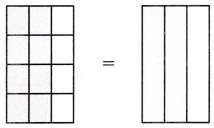(A) $$\frac{2}{3}$$
(B) $$\frac{1}{3}$$
(C) $$\frac{2}{12}$$
(D) $$\frac{4}{12}$$

Question 11.
Six of 8 stores have power drinks on sale Saturday. In simplest form, what fraction of the stores has power drinks on sale Saturday?
(A) $$\frac{2}{4}$$
(B) $$\frac{3}{4}$$
(C) $$\frac{12}{18}$$
(D) $$\frac{1}{2}$$

Question 12.
Multi-Step There arc 18 students in Jacob’s homeroom. Six students bring their lunch to school. The rest eat lunch in the cafeteria, In simplest form, which shows the fraction of students that eat lunch in the cafeteria?
(A) $$\frac{1}{3}$$
(B) $$\frac{12}{18}$$
(C) $$\frac{6}{8}$$
(D) $$\frac{2}{3}$$

TEXAS Test Prep

Eight of 24 students bought lunch today. In the simplest form, what fraction of the students bought lunch today?
(A) $$\frac{2}{3}$$
(B) $$\frac{1}{3}$$
(C) $$\frac{4}{12}$$
(D) $$\frac{6}{9}$$

### Texas Go Math Grade 4 Lesson 3.3 Homework and Practice Answer Key

Question 1.
Write $$\frac{4}{6}$$ in simplest form.
$$\frac{4}{6}$$ = $$\frac{4}{6}$$ ÷ ___________ = ___________

Question 2.
Write $$\frac{2}{4}$$ in simplest form.
$$\frac{2}{4}$$ = $$\frac{2}{4}$$ ÷ ___________ = ___________

Question 3.
Write $$\frac{3}{9}$$ in simplest form.
$$\frac{3}{9}$$ = $$\frac{3}{9}$$ ÷ ___________ = ___________

Question 4.
Write $$\frac{12}{16}$$ in simplest form.
$$\frac{12}{16}$$ = $$\frac{12}{16}$$ ÷ ___________ = ___________

Write the fraction in simplest form.

Question 5.
$$\frac{9}{12}$$ = ___________

$$\frac{4}{8}$$ = ___________

Question 7.
$$\frac{10}{12}$$ = ___________

Question 8.
$$\frac{2}{12}$$ = ___________

Question 9.
$$\frac{15}{20}$$ = ___________

Question 10.
$$\frac{3}{9}$$ = ___________

Problem Solving

Use the map for 11 and 12.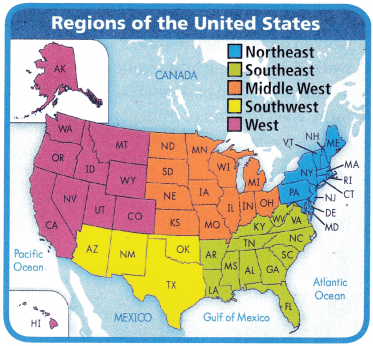Question 11.
fraction of the states in the Middle West border states in the Southeast? Is this fraction in simplest form?

Question 12.
What fraction of all the states border the Gulf of Mexico? Write the fraction in simplest form.

Lesson Check

What is the simplest form of $$\frac{20}{100}$$?
(A) $$\frac{2}{10}$$
(B) $$\frac{4}{5}$$
(C) $$\frac{10}{20}$$
(D) $$\frac{1}{5}$$

Question 14.
Twelve out of 14 players were on time for basketball practice. In the simplest form, what fraction of the players were on time for practice?
(A) $$\frac{6}{14}$$
(B) $$\frac{2}{7}$$
(C) $$\frac{6}{7}$$
(D) $$\frac{3}{14}$$

Question 15.
What is the simplest form of $$\frac{4}{24}$$ ?
(A) $$\frac{1}{4}$$
(B) $$\frac{1}{12}$$
(C) $$\frac{2}{12}$$
(D) $$\frac{1}{6}$$

Question 16.
Fifteen out of 25 students in Ms. Taylor’s class are girls. In simplest form, what fraction of the class is girls?
(A) $$\frac{3}{5}$$
(B) $$\frac{2}{5}$$
(C) $$\frac{10}{20}$$
(D) $$\frac{3}{4}$$

Multi-Step After school, 5 students worked on math homework, 3 students worked on spelling homework, and 4 students played basketball. In the simplest form, what fraction of students worked on homework?
(A) $$\frac{2}{3}$$
(B) $$\frac{3}{5}$$
(C) $$\frac{4}{8}$$
(D) $$\frac{1}{4}$$
(A) $$\frac{2}{5}$$
(B) $$\frac{5}{7}$$
(C) $$\frac{2}{3}$$
(D) $$\frac{6}{8}$$